# Verbal Reasoning - Character Puzzles

### Exercise :: Character Puzzles - Character Puzzles 1

6.

Which one will replace the question mark ?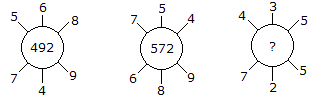A. 115 B. 130 C. 135 D. 140

Explanation:

(5 x 6 x 8) + (7 x 4 x 9) = 492

and (7 x 5 x 4) + (6 x 8 x 9) = 572

Therefore (4 x 3 x 5) + (7 x 2 x 5) = 130.

7.

Which one will replace the question mark ?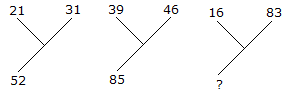A. 92 B. 72 C. 62 D. 99

Explanation:

21 + 31 = 52

and 39 + 46 = 85

Therefore, 16 + 83 = 99.

8.

Which one will replace the question mark ?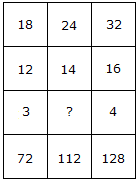A. 2 B. 3 C. 4 D. 5

Explanation:

(18 x 12)/3 = 72

and (32 x 16)/4 = 128

Therefore, (24 x 14)/? = 112

(336/?) = 112

? = (336/112)

? = 3.

9.

Which one will replace the question mark ?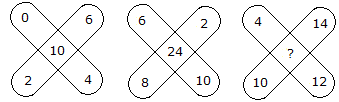A. 36 B. 48 C. 38 D. 30

Explanation:

(0 + 2 + 6 + 4) - 2 = 10

and (6 + 2 + 10 + 8) - 2 = 24

Therefore, (4 + 14 + 12 + 10) - 2 = 38.

10.

Which one will replace the question mark ?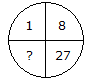A. 41 B. 64 C. 35 D. 61

Explanation:

(1)3 = 1, (2)3 = 8, (3)3 = 27

Therefore, (4)3 = 64.